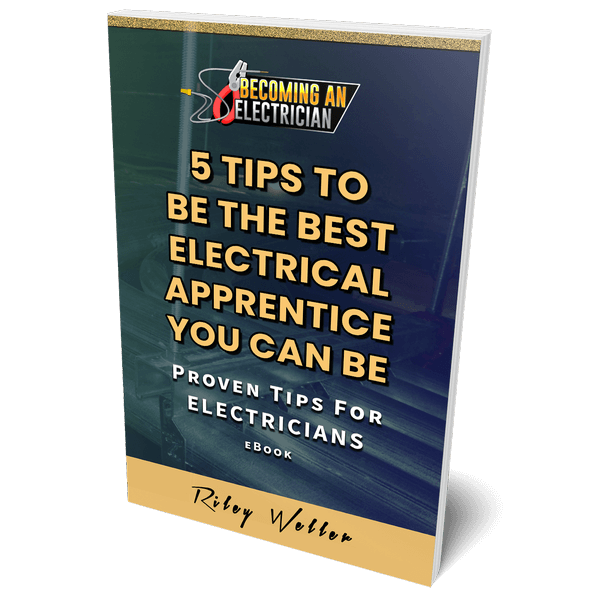# Category: Electrical Theory

After being hands-on in the trade for awhile, going to school helps electrical theory make more sense. You get to learn the code rules we have to follow as electricians like wire ampacity, pipe fill, and even the science behind electricity with magnetism, etc.

## How to Solve Parallel Circuits for Electricians

How to solve parallel circuits for electricians and parallel circuit principles. Also, how to find total parallel resistance math formula in a circuit.

## How to Solve Series Circuits for Electricians

How to solve series circuits for electricians. Series math equation examples and series circuit principles for electricians.

## What is Ohm’s Law for Electricians

Ohm’s Law is simply a math triangle electricians use to calculate Voltage, Amps, and Resistance in a SUPER EASY way. It’s all based on RESISTANCE being constant.

## What is a Power Source for Electricians

In electrical circuits we have a power source and electrical loads. A power source is what provides power to the circuit, whether it’s an AC or DC circuit, and an electrical load CONSUMES power from that power source.

## How Does a Basic Electrical Circuit Work

A basic electrical circuit works by connecting two wires (Hot and Neutral) across an electrical device (known as a “load”).## FREE BOOK!5 Tips to Be the Best Electrical Apprentice You Can Be!

Valuable tips from my apprentice years..